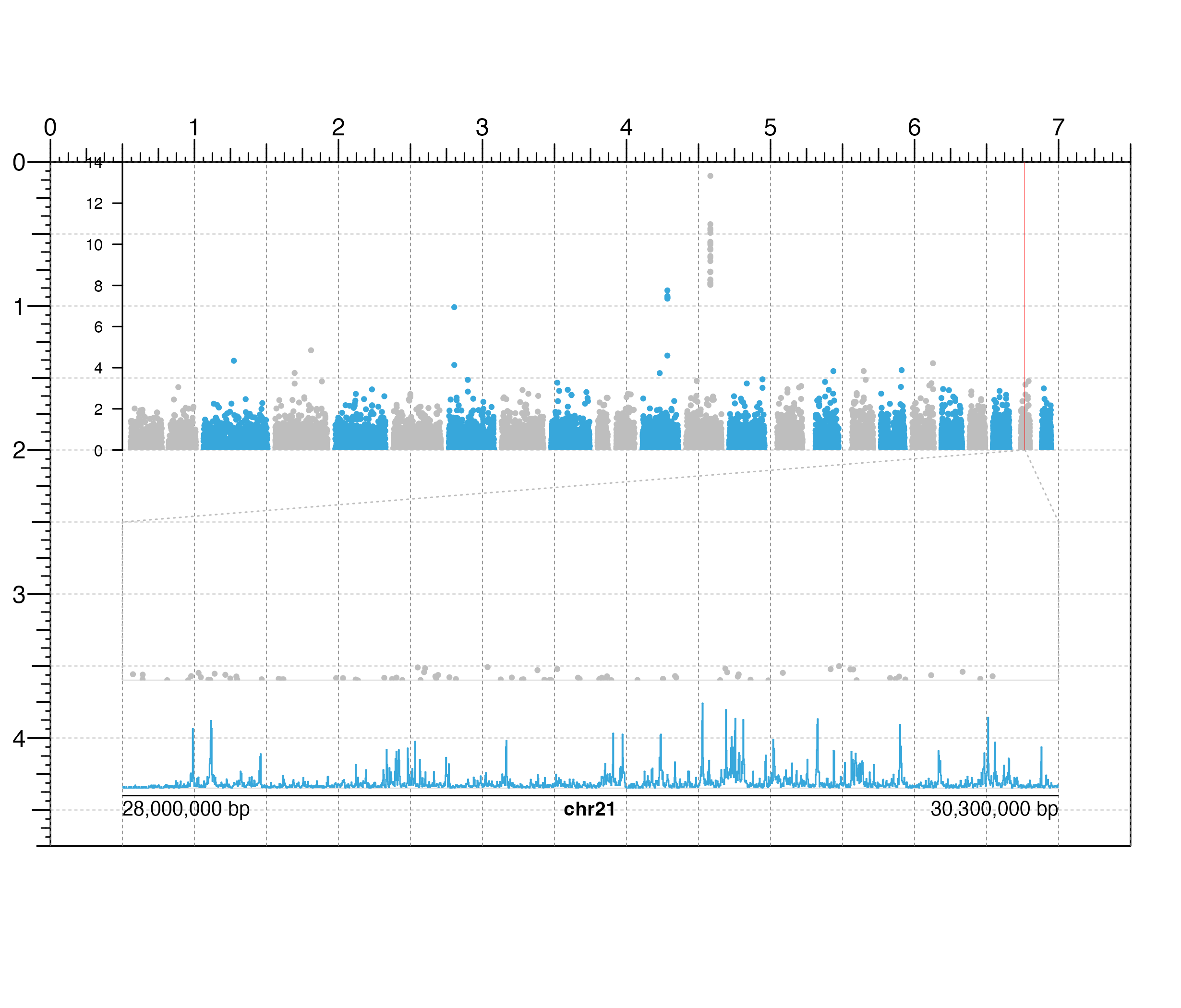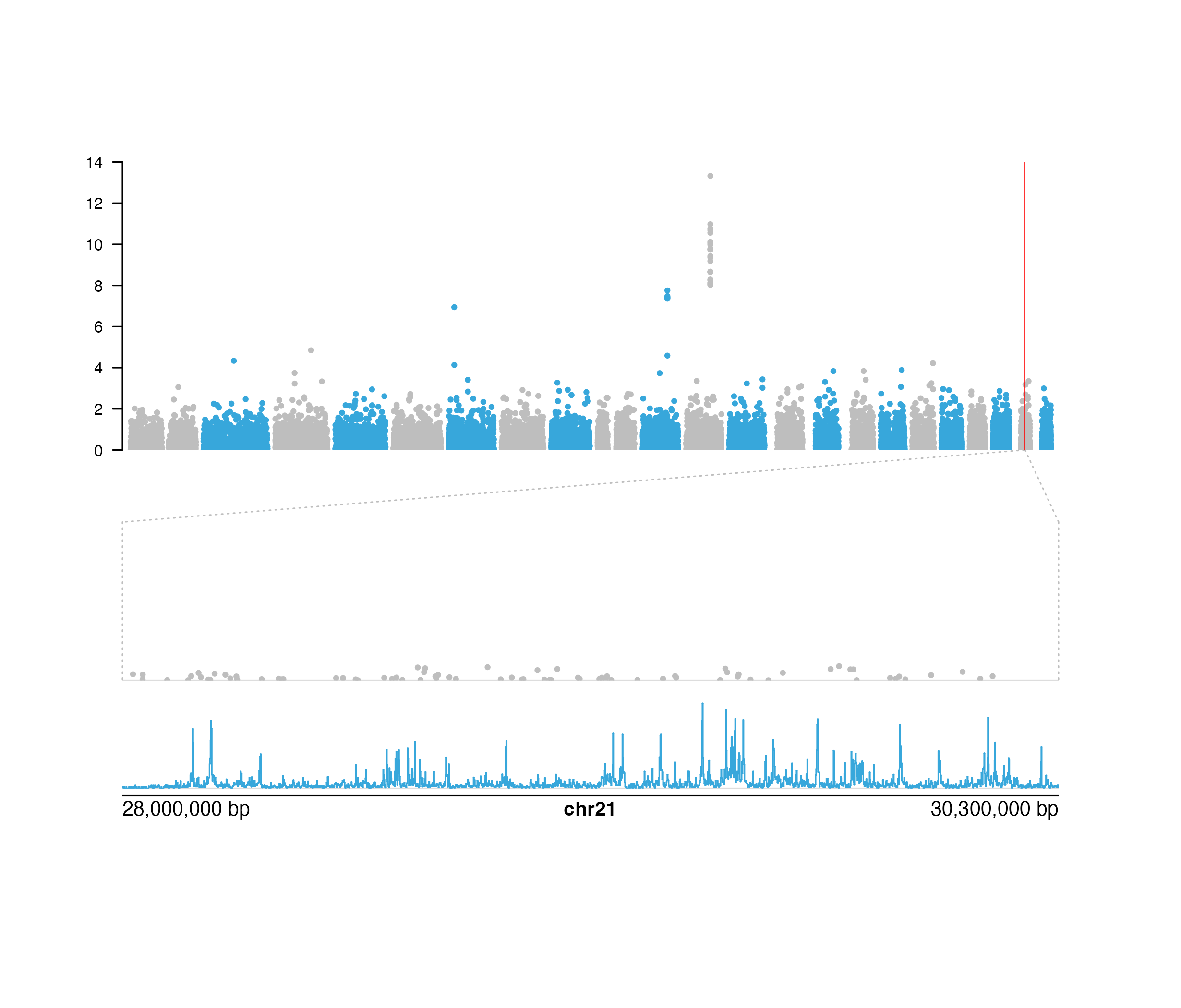Annotates zoom lines for a specified genomic region of a plot

annoZoomLines(
plot,
chrom,
chromstart = NULL,
chromend = NULL,
y0,
x1 = NULL,
y1,
extend = 0,
default.units = "inches",
linecolor = "grey",
lty = 2,
params = NULL,
...
)

## Arguments

plot

Input plot to annotate genomic region zoom lines from.

chrom

Chromosome of region to draw zoom lines from, as a string.

chromstart

Integer start position on chromosome to draw zoom lines from.

chromend

Integer end position on chromosome to draw zoom lines from.

y0

A numeric vector or unit object indicating the starting y-values of the zoom line segments. If two values are given, the first value will correspond to the left zoom line and the second value will correspond to the right zoom line.

x1

A numeric vector or unit object indicating the stopping x-values of the zoom line segments. If two values are given, the first value will correspond to the left zoom line and the second value will correspond to the right zoom line. If NULL, straight lines from zoomed genomic region will be drawn.

y1

A numeric vector or unit object indicating the stopping y-values of the zoom line segments. If two values are given, the first value will correspond to the left zoom line and the second value will correspond to the right zoom line.

extend

A numeric vector or unit object indicating the length to extend straight lines from each end of the zoom line segments. If two values are given, the first value will correspond to the top extension length and the second value will correspond to the bottom extension length. Default value is extend = 0.

default.units

A string indicating the default units to use if y0, x1, y1, or extend are only given as numerics or numeric vectors. Default value is default.units = "inches".

linecolor

A character value specifying zoom line color. Default value is linecolor = "grey".

lty

A numeric specifying zoom line type. Default value is lty = 2.

params

An optional pgParams object containing relevant function parameters.

...

Additional grid graphical parameters. See gpar.

## Value

Returns a zoom object containing relevant genomic region, placement, and grob information.

## Examples

## Create a page
pageCreate(width = 7.5, height = 4.75, default.units = "inches")

## Plot and place a Manhattan plot
library(plotgardenerData)
library("TxDb.Hsapiens.UCSC.hg19.knownGene")
data("hg19_insulin_GWAS")
manhattanPlot <- plotManhattan(
data = hg19_insulin_GWAS, assembly = "hg19",
fill = c("grey", "#37a7db"),
sigLine = FALSE,
col = "grey", lty = 2, range = c(0, 14),
x = 0.5, y = 0, width = 6.5, height = 2,
just = c("left", "top"),
default.units = "inches"
)
#> manhattan[manhattan1]
annoYaxis(
plot = manhattanPlot, at = c(0, 2, 4, 6, 8, 10, 12, 14),
axisLine = TRUE, fontsize = 8
)
#> yaxis[yaxis1]

## Annotate zoom lines for a region on chromsome 21
zoomRegion <- pgParams(
chrom = "chr21",
chromstart = 28000000, chromend = 30300000,
assembly = "hg19"
)
annoZoomLines(
plot = manhattanPlot, params = zoomRegion,
y0 = 2, x1 = c(0.5, 7), y1 = 2.5, extend = c(0, 1.1),
default.units = "inches",
lty = 3
)
#> zoom[zoom1]

## Annotate highlight region for zoom region
annoHighlight(
plot = manhattanPlot, params = zoomRegion,
y = 2, height = 2, just = c("left", "bottom"),
default.units = "inches",
fill = "red", alpha = 0.8
)
#> highlight[highlight1]

## Plot Manhattan plot data and signal track under zoom lines
manhattanPlotZoom <- plotManhattan(
data = hg19_insulin_GWAS,
fill = "grey",
sigLine = FALSE,
baseline = TRUE,
params = zoomRegion, range = c(0, 14),
x = 0.5, y = 2.6,
width = 6.5, height = 1
)
#> manhattan[manhattan2]
data("IMR90_ChIP_H3K27ac_signal")
signalPlot <- plotSignal(
data = IMR90_ChIP_H3K27ac_signal, params = zoomRegion,
range = c(0, 45),
x = 0.5, y = "b0.1",
width = 6.5, height = 0.65,
just = c("left", "top"),
default.units = "inches"
)
#> signal[signal1]

## Plot genome label
plotGenomeLabel(
chrom = "chr21",
chromstart = 28000000, chromend = 30300000,
assembly = "hg19",
x = 0.5, y = 4.4, length = 6.5,
default.units = "inches"
)
#> genomeLabel[genomeLabel1]## Hide page guides
pageGuideHide()